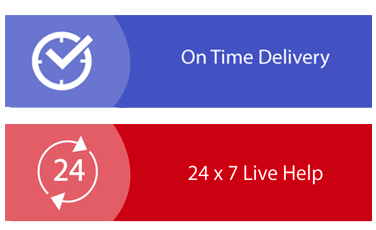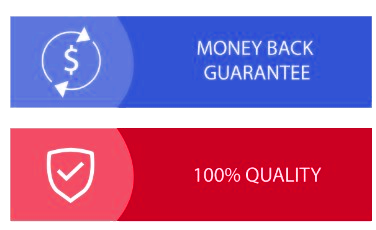× Limited Time Offer ! FLAT 30-40% off - Grab Deal Before It’s Gone. Order Now

## STA201 Business Statistics Assignment Sample

### Assessment - Analytics Assignment

Individual/Group - Individual
Length - 1000 Words

Learning Outcomes - The Subject Learning Outcomes demonstrated by successful completion of the task below include:

a) Produce, analyse, and present data graphically and numerically, and perform statistical analysis of central tendency and variability.

b) Apply inferential statistics to draw conclusions about populations, including confidence levels, hypothesis testing, analysing variance and comparing with benchmarks in decision making processes.

c) Measure uncertainty, including continuous and discrete probability and sampling distributions to select appropriate methods of data analysis.

d) Apply parametric tests and analysis techniques to determine causation and forecasting to assist decision making.

e) Utilise technology to analyse and manipulate data and present findings to peers and other stakeholders.
Submission Due by 11:55 pm AEST/AEDT Sunday end of Module 6.2 (Week 12).

Weighting - 30%

### Assessment Tasks for assignment help

1. Prepare a summary statistics table and discuss the central tendency and variations for all variables.

2. Plot the dependent variable (house price), against each independent variable using scatter plot/dot function in Excel. Comment on the strength and the nature of the relationship between the dependent and the independent variables.

3. Generate a multiple regression summary output. Using the information on regression output, state the multiple regression equation.

4. Interpret the meaning of slope coefficients of independent variables.

5. Interpret the R 2 and adjusted R 2 of your model.

6. Conduct a test for significance of your overall multiple regression model at the 0.05 level of significance. You must state your null and alternative hypotheses.

7. At the 0.05 level of significance, determine whether each independent variable makes a significant contribution to the regression model. Based on these results, indicate the independent variables to include in this model.

8. Construct a 95% confidence interval estimate of population slope of house prices withproperty size. Interpret your results.

9. Construct a 95% confidence interval estimate of population slope of house prices with distance to nearest train station. Interpret your results.

10. Choose one of the houses currently advertised for sale in your chosen suburb (the one to collect the data of). Make sure to choose a house whose asking price is also advertised. Predict the price of the house using the regression equation you generated in part 3 and values of the independent variables as advertised. Compare the predicted price with the asking price.

## Solution

As per the table 1 in appendix, it can be seen that mean value of sale price is \$465542 with median \$422500 and mode \$420000. Hence, the average sales price is \$465542, whereas most of the property price is \$420000, thus the data is not normally distributed. Standard deviation is 141997.29 and sample variance is 20163229016.33. Thus, the housing sales price has very high variability in it. Kurtosis value of 3.09 signifies the fact that there are outliers and range of \$790000 also demonstrates maximum and minimum housing price has very high difference in it (Bono et al., 2019). Mean value of bedroom is 3.78 with mode 4.

Average bedroom number is 3.78 whereas most of the property has 4 bedrooms. Standard deviation and sample variance is 0.51 and .26 respectively. Thus, the variation in data of bedroom is low. Kurtosis value is 2.42 that showcase there is low number of outliers with negative skewness that has right tail. Bathroom average count is 1.86 with mode 2. Thus, most of the houses has 2 bathrooms with average value of bathroom 1.86. Low standard deviation of 0.45 and sample variance of 0.20 showcase bathroom data has limited variability. Kurtosis value is 1.39 with range of 2 showcase there is low to moderate variability in the bathroom count data.

Property size has mean value of 791.40 m2 with mode752 m2 and median of 751 m2. Thus, most of the property size is 751 m2 with average value of 791.40 m2. Standard deviation and sample variance is very high that showcase property size has high variability. Kurtosis value of 34.49 and range of 3837 showcase there is very high variability in property size data. As per skewness of 5.37, data is highly skewed, and it has left tail. Average distance of property from metro station is .46 km, however, as per mode, most of the property has average distance of .43 km. Thus, the data is normally distributed, and it has low variability with standard deviation of .29 and sample variance of .08. Kurtosis of 4.05 demonstrates there is outliers and range signifies that the data has low variability in it.

### Task 2: plotting dependent variables against independent variable: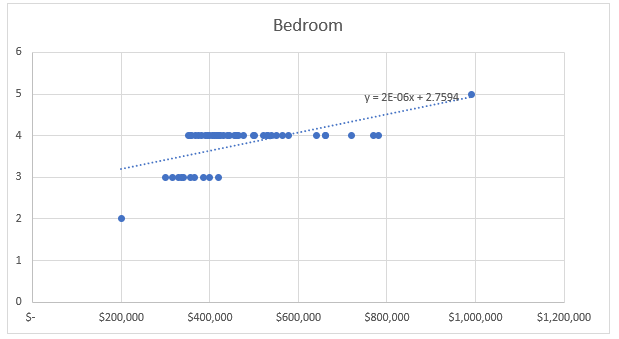Figure 1: Association between number bedroom and property price

Figure 1 demonstrates the association between bedroom and property price. Though the plots did not showcase anything clearly, however, an upward sloping trend line demonstrated a positive association between the variables (Schober et al., 2018). Positive association demonstrates increase in number of bedrooms, increase the housing price.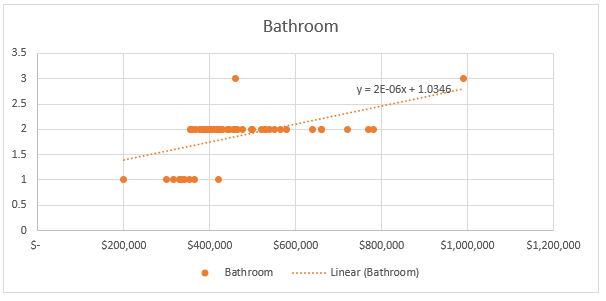Figure 2: Association between bathroom number and property price

As per the figure 2 it can be seen that there is also an upward trend in price as the number of bathroom increases. Hence, the association is positive, however the degree of association is not clear from here.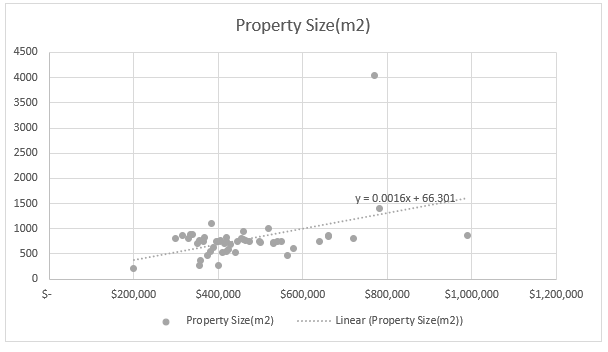Figure 3: Association between property size and property price

Figure 3 demonstrated as the property size increase; price of property also increase. However, the plots are not scattered demonstrating, as the property size increase price of property does not increase moderately. Hence, though the trend line showcase a positive association between two values, yet the degree of association is not very high.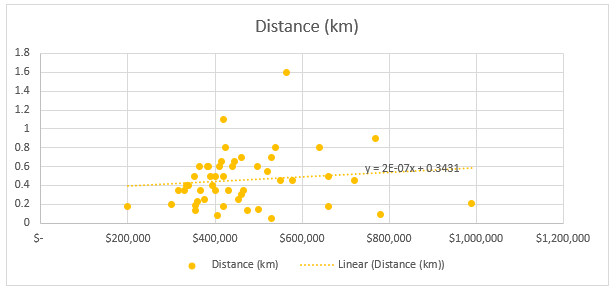Figure 4: Association between distance from metro and property price

Figure 4 demonstrates a positive association between the distance from metro and property price. Hence, with rise in distance of property from metro, price tends to rise. However, as the distance increase, housing price does not increase proportionately as per plot demonstrating a weak association.

To develop model that can predict the housing price based on independent variables, multiple regression has been done here. As per table 2 in appendix, following regression model can be mentioned:

Y = -169462.44 + 104230.45 * X1 + 80772.80 * X2 + 100.18 * X3 + 25196.60 * X4 [Where, Y is property price, X1 is bedroom number, X2 is bathroom number, X3 is property size and X4 is distance from metro]

### Task 4: Slope coefficient interpretation:

As per table 2, slope coefficient of bedroom is 104230.45 which mean, change in 1 unit of bedroom can lead the property price to increase by \$104230.45. Slope coefficient of bathroom demonstrates 1 unit of bathroom addition can lead to rise in house price by \$80772.80. As per property size slope coefficient, 1 m2 rise in property size increase the property price by \$100.18. Distance slope coefficient, 1 km rise in distance can increase the housing price by \$25196.60.

R square value of the model is 0.53, which mean independent variables can explain 53% of variability of the data in dependent variable. Adjusted R Square demonstrates how additional independent variable can change the predictability of the model for dependent variable (Sharma et al., 2021). Adjusted R Square value of 0.49, means addition of exploratory variables can actually fails to make the model more significant in predicting variability of data.

### Task 6: Test of significance of model:

Null hypothesis: H0: Fit of intercept only model is equal to predicted model

Alternative hypothesis: H1: Fit of intercept only model is significantly reduced compared to predicted model
As per the ANOVA in table, F value is 0.00, which is lower than critical value of 0.05. Hence, the model is a good fit model and null hypothesis is accepted here.

### Task 7: Test of significance of independent variable:

At 0.05 level of significance, as per table 2, only bedroom and property size seems to significantly influence the property price as their p value is 0.02 and 0.00 respectively. For other exploratory variables, p value is 0.1 (for bathroom) and 0.62 (for distance) which is higher than critical value of 0.05. Thus, bathroom and distance cannot significantly influence the property price.

Considering the significance of bedroom and property size, these factors can only be included in the regression model; thus, the revised regression model is as follows:

Y = -169462.44 + 104230.45 * X1 + 100.18 * X3

### Task 8: 95% confidence interval of property size:

Confidence interval = b1 ± t(1-α)/2, n-2 * se(b1)
At 95% confidence interval, t(1-α)/2, n-2 = 2.011
b1 = 100.18
se(b1) = 28.92
Confidence interval = 100.18 ± 2.011 * 28.92 = 58.13
Confidence interval = 100.18 ± 58.13 = 158.13, 42.05

So, with 95% confidence interval, one m2 change in property size change the housing price between \$158.13 and \$42.05.

### Task 9: 95% Confidence interval of distance:

Confidence interval = b1 ± t(1-α)/2, n-2 * se(b1)
At 95% confidence interval, t(1-α)/2, n-2 = 2.011
b1 = 25196.60
se(b1) = 51167.47

Confidence interval = 25196.60 ± 2.011 * 51167.47 = 128094.38, -77701.18

So, with 95% confidence interval it can be said that with 1 km change in distance, housing price change between \$128094.38 and -\$77701.18.

### Task 10: Estimation of House Price:

House price at Clayton with 3-bedroom, 1 bathroom, 191 m2 property size and .5 km distance to metro:

Y = -169462.44 + 104230.45 * 3 + 100.18 * 191 = \$162363.61

Hence, the estimated property price at Clayton would be \$162363.31

### Reference:

Bono, R., Arnau, J., Alarcón, R., & Blanca, M. J. (2019). Bias, precision, and accuracy of skewness and kurtosis estimators for frequently used continuous distributions. Symmetry, 12(1), 19. https://www.mdpi.com/2073-8994/12/1/19/pdf

Schober, P., Boer, C., & Schwarte, L. A. (2018). Correlation coefficients: appropriate use and interpretation. Anesthesia & Analgesia, 126(5), 1763-1768. https://journals.lww.com/anesthesia-analgesia/Fulltext/2018/05000/Correlation_Coefficients___Appropriate_Use_and.50.aspx

Sharma, P. N., Shmueli, G., Sarstedt, M., Danks, N., & Ray, S. (2021). Prediction?oriented model selection in partial least squares path modeling. Decision Sciences, 52(3), 567-607. http://www.tara.tcd.ie/bitstream/handle/2262/93731/Predictive%20Model%20Selection_DSJ_Author_Copy1.pdf?sequence=1

Fill the form to continue reading